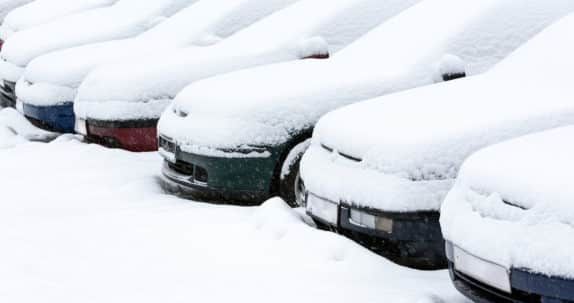# Deicing Parking Lots: How To Calculate Material## 09 Oct Deicing Parking Lots: How To Calculate Material

To determine the amount of deicer for a parking lot, you need to do a few simple calculations based on area. You will also need a few simple tools to ensure accuracy. You can get an estimated distance by utilizing google maps. This works by using the scale of the map to measure the area of a projected job. For smaller deicing jobs, the best tool is a measuring wheel. A wheel allows to more accurate measuring and closer product estimation.

Here are some simple ways to calculate the area of your parking lot:

• Ask your customer for a scaled map of the facility. A customer may have maps or prints of their property from when it was constructed. This would be the most accurate measurement without having to measure it yourself.
• Ask the customer for the size of the area they want treated. You should always double check this measurement for accuracy.
• Measure the area yourself to be able to provide the most accurate measurement.

If you are measuring an area yourself, a quick review of high school geometry is all that is necessary. You will need to understand how to calculate the area of three basic shapes: a square or rectangle, a triangle and a circle.

• A square or rectangle: Multiply the length times the width for area.
• A triangle: Multiply the length times with width and divide by two for the area.
• A circle: Calculate the distance from the counterpoint of the circle to the edge (circumference) for the radius. The radius squared times 3.14 (pi) results in the area of the circle. You can also use this online tool.

These calculations can also be used for dust control projects in the summer. Happy calculating!